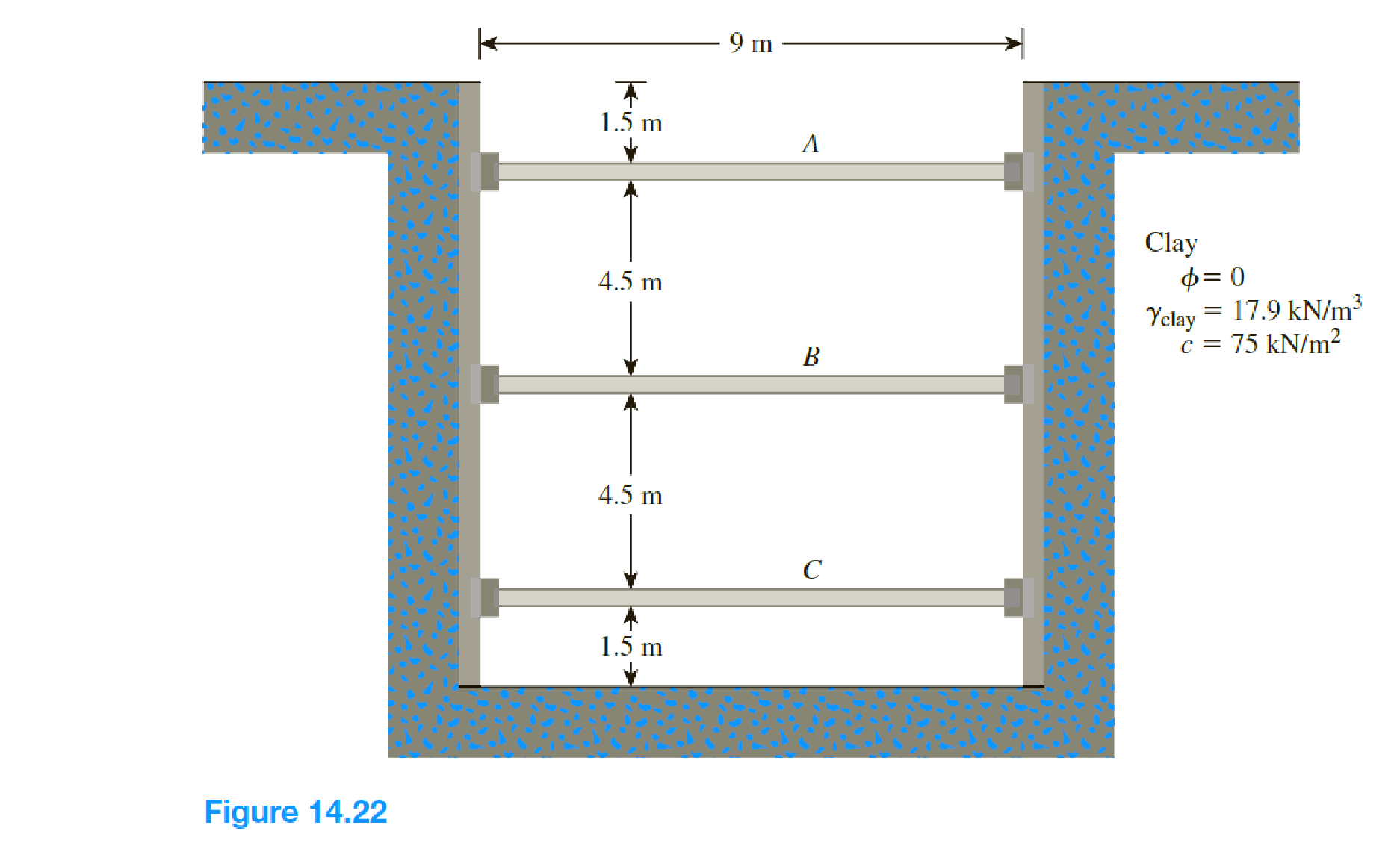Chapter 14, Problem 14.15PPrinciples of Geotechnical Enginee...

9th Edition
Braja M. Das + 1 other
ISBN: 9781305970939

Solutions

Chapter
SectionPrinciples of Geotechnical Enginee...

9th Edition
Braja M. Das + 1 other
ISBN: 9781305970939
Textbook Problem

The cross section of a braced cut supporting a sheet pile installation in a clay soil is shown in Figure 14.22. Given: H = 12 m, γclay = 17.9 kN/m3, ϕ = 0, c = 75 kN/m2, and the center-to-center spacing of struts in plan view, s = 3 m. a. Using Peck’s empirical pressure diagrams, draw the earth-pressure envelope. b. Determine the strut loads at levels A, B, and C.(a)

To determine

Draw the earth-pressure envelope using Peck’s empirical pressure diagram.

Explanation

Given information:

The unit weight γclay of the clay is 17.9kN/m3.

The soil friction angle ϕ is 0.

The cohesion c is 75kN/m2.

The depth of level A is z=1.5m.

The depth of level B is x=4.5m.

The depth of level C is x=4.5m.

The depth below level C is z=1.5m.

The total depth H is 12 m.

Calculation:

Determine the value of value of γclayHc.

Substitute 17.9kN/m3 for γclay, 12 m for H, and 75kN/m2 for c.

γclayHc=17

To determine

Find the design strut loads at level A, B, and C.

Still sussing out bartleby?

Check out a sample textbook solution.

See a sample solution

The Solution to Your Study Problems

Bartleby provides explanations to thousands of textbook problems written by our experts, many with advanced degrees!

Get Started

Explain how flow of traffic is related to density and average speed.

Engineering Fundamentals: An Introduction to Engineering (MindTap Course List)

Briefly describe the principle of laser machining.

Precision Machining Technology (MindTap Course List)

Define the term topology, and draw a sketch of each wired and wireless network topology.

Systems Analysis and Design (Shelly Cashman Series) (MindTap Course List)

Which VLAN on a switch manages untagged frames?

Network+ Guide to Networks (MindTap Course List)

What should you do if you have to leave a piece of hot metal unattended?

Welding: Principles and Applications (MindTap Course List)

List jobs that require, or jobholders who may benefit from, obtaining an operating system certification.

Enhanced Discovering Computers 2017 (Shelly Cashman Series) (MindTap Course List)